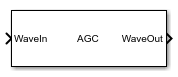# AGC

Automatically adjusts gain to maintain output waveform amplitude

• Library:
• SerDes Toolbox / Datapath Blocks## Description

The AGC block applies an adaptive variable gain to the input waveform to achieve a desired RMS output voltage. Averaging the RMS voltage over a specified number of symbols, AGC performs automatic gain control (AGC) by increasing or decreasing the gain, or keeping the gain constant.

## Ports

### Input

expand all

Input baseband signal. The input signal can be a sample-by-sample signal specified as a scalar, or an impulse response vector signal.

Data Types: `double`

### Output

expand all

Gain adjusted output signal. If the input signal is a sample-by-sample signal specified as a scalar, the output is also scalar. If the input signal is an impulse response vector signal, the output is also a vector.

Data Types: `double`

## Parameters

expand all

AGC operating mode:

• `Off`AGC is bypassed, the input waveform remains unchanged.

• `On`AGC adjusts gain of input waveform to maintain Target RMS voltage in output waveform.

#### Programmatic Use

• Use `get_param(gcb,'Mode')` to view the current AGC Mode.

• Use `set_param(gcb,'Mode',value)` to set AGC to a specific Mode.

Desired RMS voltage of the output waveform, specified as a real scalar in the range [0.003, 10] in volts.

#### Programmatic Use

• Use `get_param(gcb,'TargetRMSVoltage')` to view the current value of Target RMS voltage (V).

• Use `set_param(gcb,'TargetRMSVoltage',value)` to set Target RMS voltage (V) to a specific value.

Data Types: `double`

Maximum allowed AGC gain, specified as a positive real scalar. Maximum gain provides a stable startup of the adaptive algorithm.

#### Programmatic Use

• Use `get_param(gcb,'MaxGain')` to view the current value of Maximum gain.

• Use `set_param(gcb,'MaxGain',value)` to set Maximum gain to a specific value.

Data Types: `double`

Averaging length, specified as a positive real integer. Averaging length defines the number of symbol over which the RMS calculation of the input signal is made.

#### Programmatic Use

• Use `get_param(gcb,'AveragingLength')` to view the current value of Averaging length.

• Use `set_param(gcb,'AveragingLength',value)` to set Averaging length to a specific value.

Data Types: `double`

Choose which parameters to be included in IBIS-AMI models. By default, both parameters are selected.

If you deselect a parameter, the parameter is removed from the AMI files, effectively hard-coding the parameter to its current value. For example, if Target RMS voltage (V) is set to `0.5` and you clear the check box for Target RMS voltage under IBIS-AMI parameters, the value of Target RMS voltage (V) is hard-coded to `0.5` V.